### Home > A2C > Chapter Ch13 > Lesson 13.1.1 > Problem13-23

13-23.
1. A jar contains five red, four white, and three blue balls. If three balls are randomly selected, find the probability of choosing: Homework Help ✎

1. Two red and one white.

2. Three white.

3. One of each color.

4. All the same color.

5. One red and two white.

6. Two of one color and one of another.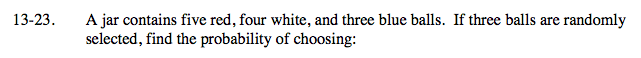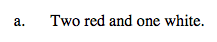$\frac{_5C_2 \cdot _4C_1}{_{12}C_5}=\frac{2}{11}$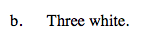See part (a).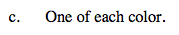See part (a).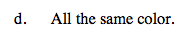$\frac{_5C_3 + _4C_3 + _3C_3}{_{12}C_3}=\frac{3}{44}$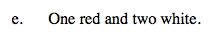See part (a).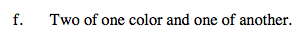How can the probabilities from parts (c) and (d)